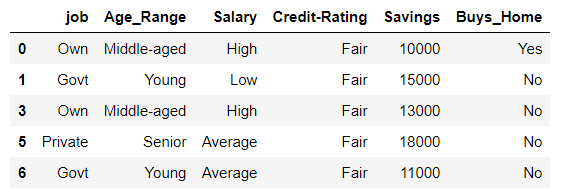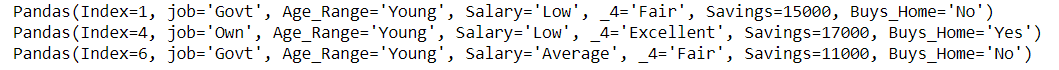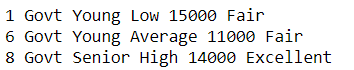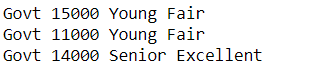# Select rows that contain specific text using Pandas

While preprocessing data using pandas dataframe there may be a need to find the rows that contain specific text. In this article we will discuss methods to find the rows that contain specific text in the columns or rows of a dataframe in pandas.

Dataset in use:

### Method 1 : Using contains()

Using the contains() function of strings to filter the rows. We are filtering the rows based on the ‘Credit-Rating’ column of the dataframe by converting it to string followed by the contains method of string class. contains() method takes an argument and finds the pattern in the objects that calls it.

Example:

## Python3

 `# importing pandas as pd ` `import` `pandas as pd ` ` `  `# reading csv file ` `df ``=` `pd.read_csv(``"Assignment.csv"``) ` ` `  `# filtering the rows where Credit-Rating is Fair ` `df ``=` `df[df[``'Credit-Rating'``].``str``.contains(``'Fair'``)] ` `print``(df) `

Output :Rows containing Fair as Savings

### Method 2 : Using itertuples()

Using itertuples() to iterate rows with find to get rows that contain the desired text. itertuple method return an iterator producing a named tuple for each row in the DataFrame. It works faster than the iterrows() method of pandas.

Example:

## Python3

 `# importing pandas as pd ` `import` `pandas as pd ` ` `  `# reading csv file ` `df ``=` `pd.read_csv(``"Assignment.csv"``) ` ` `  `# filtering the rows where Age_Range contains Young ` `for` `x ``in` `df.itertuples(): ` `    ``if` `x[``2``].find(``'Young'``) !``=` `-``1``: ` `        ``print``(x) `

Output :Rows with Age_Range as Young

### Method 3 : Using iterrows()

Using iterrows() to iterate rows with find to get rows that contain the desired text. iterrows() function returns the iterator yielding each index value along with a series containing the data in each row. It is slower as compared to the itertuples because of lot of type checking done by it.

Example:

## Python3

 `# importing pandas as pd ` `import` `pandas as pd ` ` `  `# reading csv file ` `df ``=` `pd.read_csv(``"Assignment.csv"``) ` ` `  `# filtering the rows where job is Govt ` `for` `index, row ``in` `df.iterrows(): ` `    ``if` `'Govt'` `in` `row[``'job'``]: ` `        ``print``(index, row[``'job'``], row[``'Age_Range'``], ` `              ``row[``'Salary'``], row[``'Savings'``], row[``'Credit-Rating'``]) `

Output :Rows with job as Govt

### Method 4 : Using regular expressions

Using regular expressions to find the rows with the desired text. search() is a method of the module re. re.search(pattern, string): It is similar to re.match() but it doesn’t limit us to find matches at the beginning of the string only. We are iterating over the every row and comparing the job at every index with ‘Govt’ to only select those rows.

Example:

## Python3

 `# using regular expressions ` `from` `re ``import` `search ` ` `  `# import pandas as pd ` `import` `pandas as pd ` ` `  `# reading CSV file ` `df ``=` `pd.read_csv(``"Assignment.csv"``) ` ` `  `# iterating over rows with job as Govt and printing ` `for` `ind ``in` `df.index: ` `    ``if` `search(``'Govt'``, df[``'job'``][ind]): ` `        ``print``(df[``'job'``][ind], df[``'Savings'``][ind], ` `              ``df[``'Age_Range'``][ind], df[``'Credit-Rating'``][ind]) `

Output :Rows where job is Govt

Whether you're preparing for your first job interview or aiming to upskill in this ever-evolving tech landscape, GeeksforGeeks Courses are your key to success. We provide top-quality content at affordable prices, all geared towards accelerating your growth in a time-bound manner. Join the millions we've already empowered, and we're here to do the same for you. Don't miss out - check it out now!